Question

# Please answer all. The displacement vectors A and B shown in the figure below both have...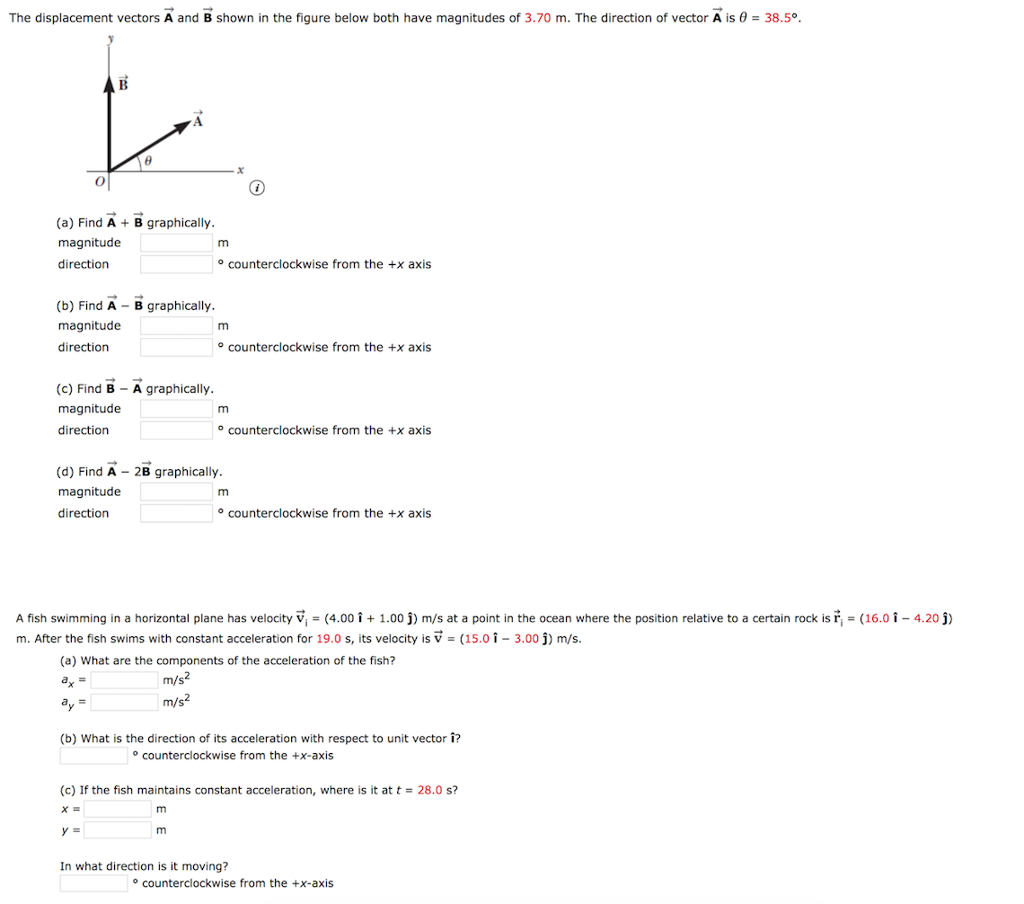Please answer all.

The displacement vectors A and B shown in the figure below both have magnitudes of 3.70 m. The direction of vector A is θ = 38.5° (a) Find A B graphically magnitude direction o counterclockwise from the +x axis (b) Find A B graphically magnitude direction o counterclockwise from the +x axis (c) Find B A graphically magnitude direction o counterclockwise from the +x axis (d) Find A 2B graphically magnitude direction o counterclockwise from the +x axis A fish swimming in a horizontal plane has velocity vi = (4.00 Î + 1.00 j) m/s at a point in the ocean where the position relative to a certain rock is ri = (16.0-4.20j) m. After the fish swims with constant acceleration for 19.0 s, its velocity is V (15.0 1-3.00j) m/s. (a) What are the components of the acceleration of the fish? m/s m/s2 (b) What is the direction of its acceleration with respect to unit vector i? o counterclockwise from the +x-axis (c) If the fish maintains constant acceleration, where is it at t28.0 s? In what direction is it moving? o counterclockwise from the +x-axis

This question is based on the concept of

1. Resolution of the vectors

2. Magnitude of resultant vector

3. Direction of the resultant vector

The detailed solution is described below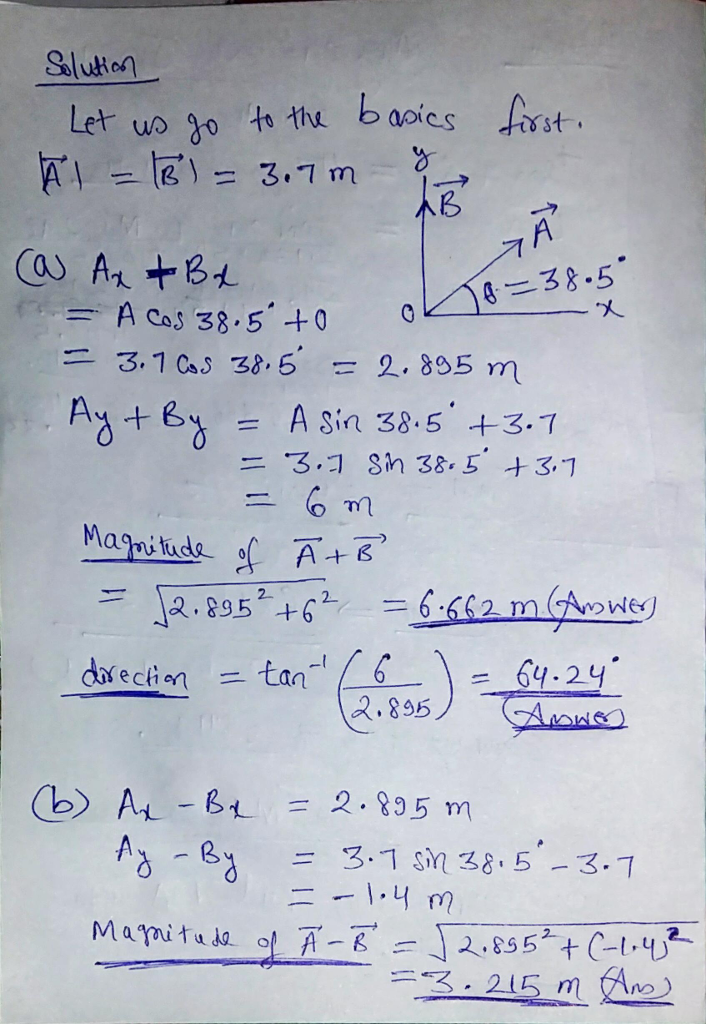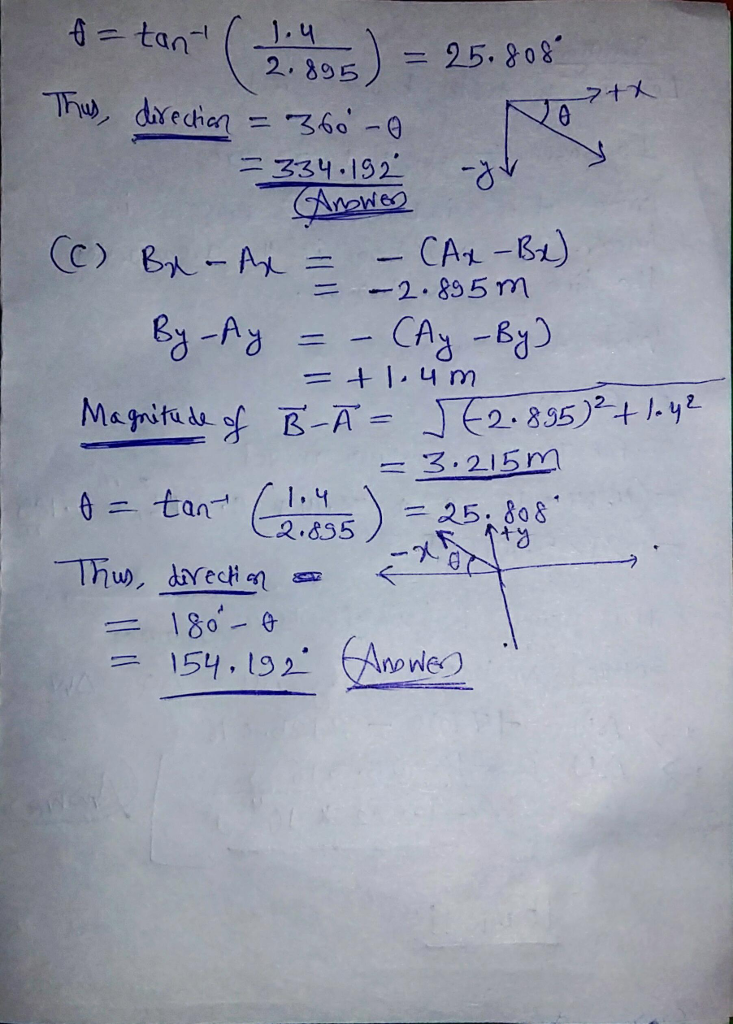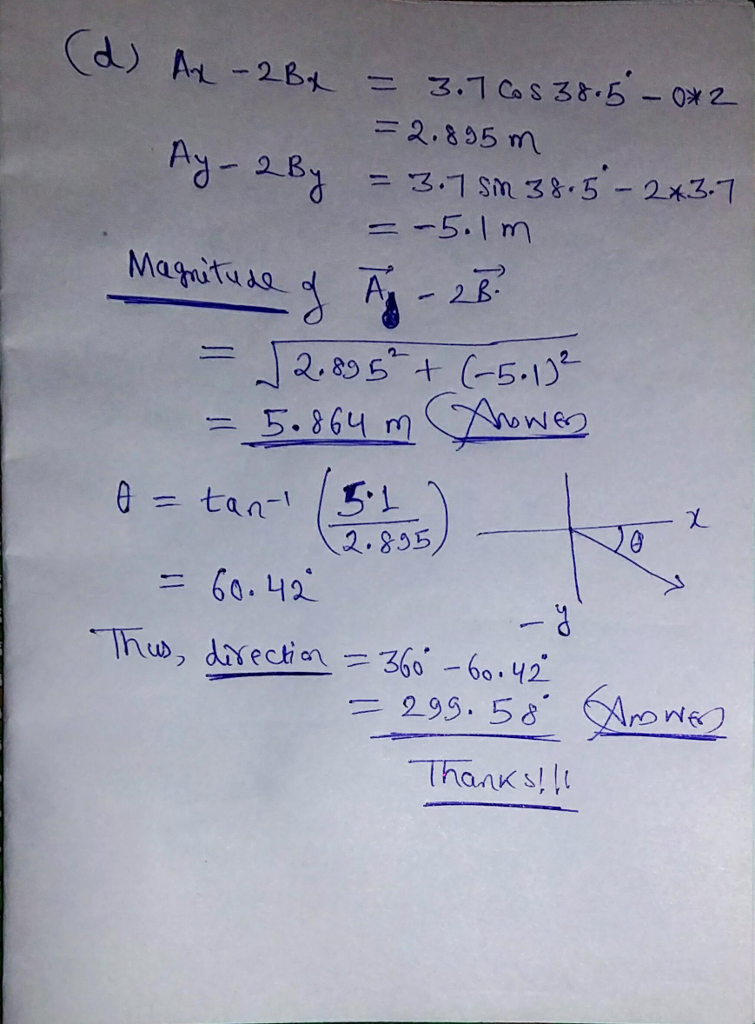#### Earn Coins

Coins can be redeemed for fabulous gifts.

Similar Homework Help Questions
• ### Please help! A fish swimming in a horizontal plane has velocity v = (4.00 i +...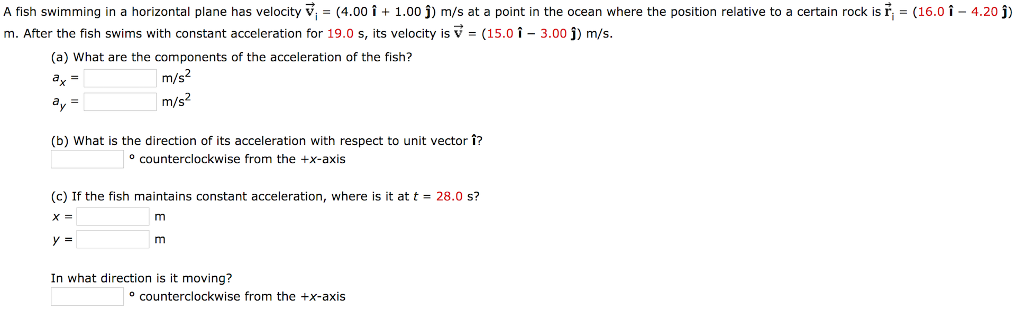Please help! A fish swimming in a horizontal plane has velocity v = (4.00 i + 1.00 j) m/s at a point in the ocean where the position relative to a certain rock is ri = (16.0-4.20 j) m. After the fish swims with constant acceleration for 19.0 s, its velocity is V (15.0 1-3.00 j) m/s. (a) What are the components of the acceleration of the fish? m/s m/s (b) What is the direction of its acceleration with respect...

• ### The displacement vectors A and B shown in the figure below both have magnitudes of 2.15...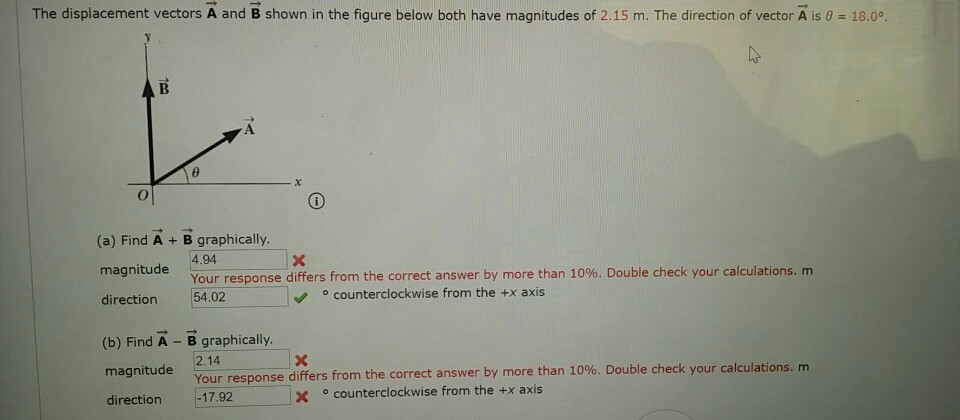The displacement vectors A and B shown in the figure below both have magnitudes of 2.15 m. The direction of vector A is θ-18.0。 (a) Find A + B graphically magnitude direction 54.02 (b) Find A - B graphically. magnitude 214 4.94 Your response differs from the correct answer by more than 10%. Double check your calculations, m ° counterclockwise from the +x axis Your response differs from the correct answer by more than 10%. Double check your calculations. m...

• ### A fish swimming in a horizontal plane has velocity v with arrowi = (4.00 i +...

A fish swimming in a horizontal plane has velocity v with arrowi = (4.00 i + 1.00 j) m/s at a point in the ocean where the position relative to a certain rock is r with arrowi = (14.0 i − 4.20 j) m. After the fish swims with constant acceleration for 19.0 s, its velocity is v with arrow = (15.0 i − 3.00 j) m/s. (a) What are the components of the acceleration of the fish? ax =...

• ### Help! A fish swimming in a horizontal plane has velocity V (4.00 i1.00 j) m/s at...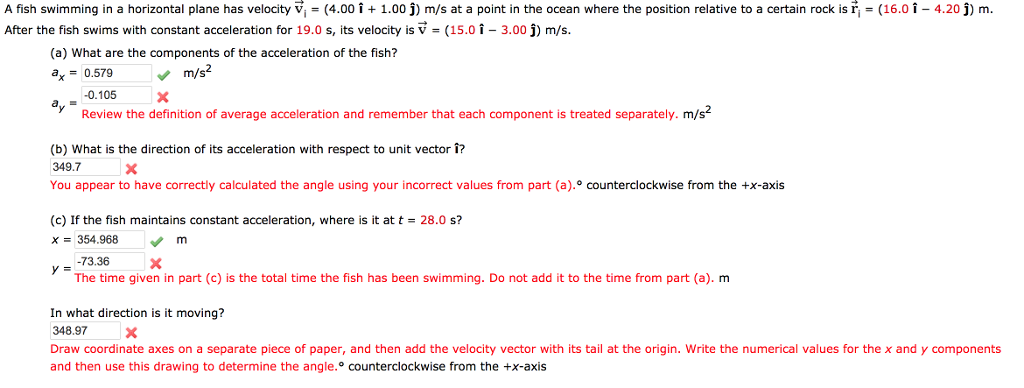Help! A fish swimming in a horizontal plane has velocity V (4.00 i1.00 j) m/s at a point in the ocean where the position relative to a certain rock is fter the fish swims with constant acceleration for 19.0 s, its velocity is V (15.0 1-3.00 j) m/s (16.0 1 4.20) m (a) What are the components of the acceleration of the fish? ax0.579 m/s2 0.105X Review the definition of average acceleration and remember that each component is treated separately....

• ### 5. + 0/1 points Previous Answers SerPSE 10 4.2.OP.005.MI. My Notes A fish swimming in a...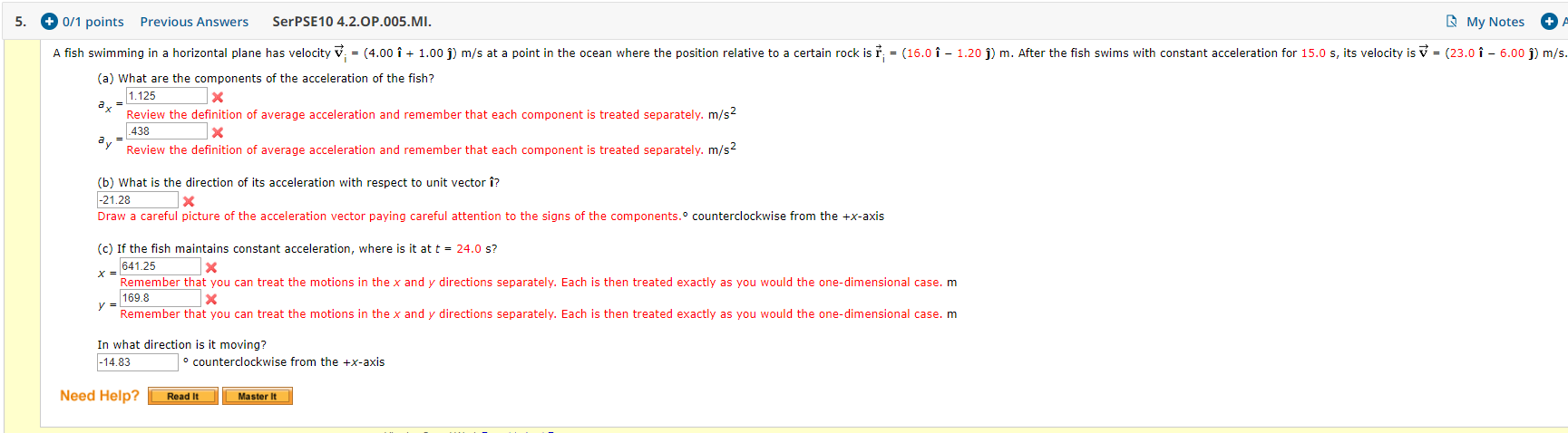5. + 0/1 points Previous Answers SerPSE 10 4.2.OP.005.MI. My Notes A fish swimming in a horizontal plane has velocity v = (4.00 i + 1.00 )) m/s at a point in the ocean where the position relative to a certain rock is † ; = (16.0 î - 1.20 j) m. After the fish swims with constant acceleration for 15.0 s, its velocity is v = (23.0 î - 6.00 ) m/s. (a) What are the components of the...

• ### A fish swimming in a horizontal plane has velocity v.- 4.00 + 1.00 j m s...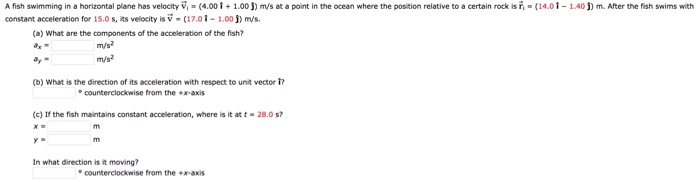A fish swimming in a horizontal plane has velocity v.- 4.00 + 1.00 j m s at a point in the ocean where the post on relative to a certain rock sn· constant acceleration for 15.0 s, its velocity is v (01 1.00j) m/s. 14,0 1-140 m. After the sh swims wit (a) What are the components of the acceleration of the fish? ay (b) What is the direction of its acceleration with respect to unit vector ? ecounterclockwise from...

• ### If the number is a decimal please include 4 digits past the decimal point if available....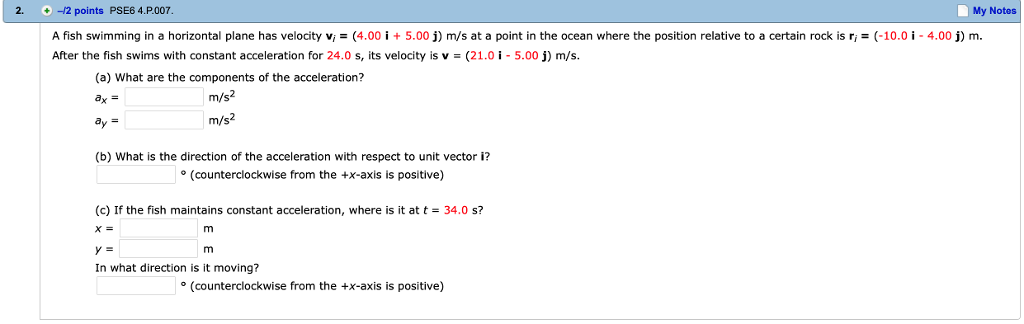If the number is a decimal please include 4 digits past the decimal point if available. 2. -12 points PSE6 4.P.007 My Notes A fish swimming in a horizontal plane has velocity vi = (4.00 İ + 5.00 j) m/s at a point in the ocean where the position relative to a certain rock is ri = (-10.01-4.00 j) m. After the fish swims with constant acceleration for 24.0 s, its velocity is v-(21.0-5.00) m/s. (a) What are the components...

• ### A fish swimming in a horizontal plane has velocity m/s at a point in a the...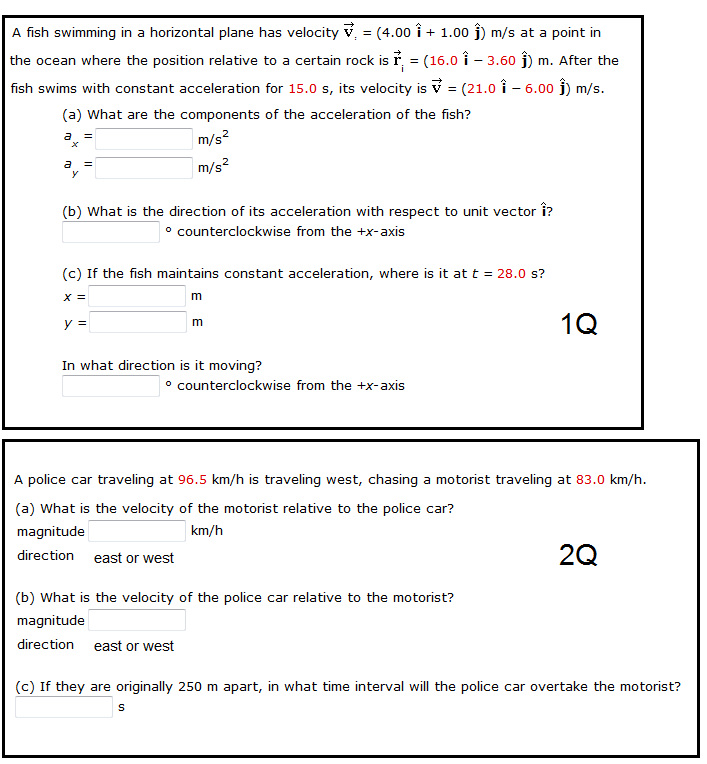A fish swimming in a horizontal plane has velocity m/s at a point in a the ocean where the position relative to a certain rock is m. After the fish swims with constant acceleration for 15.0 s, its velocity is m/s. What are the components of the acceleration of the fish? What is the direction of its acceleration with respect to unit vector ? counterclockwise from the +x-axis If the fish maintains constant acceleration, where is it at t =...

• ### Each of the displacement vectors [vector A] and [vector B] shown in the figure below has...

Each of the displacement vectors [vector A] and [vector B] shown in the figure below has a magnitude of 3.00 m. Find the following values graphically. Report all angles counterclockwise from the positive x axis. (a) [vector A] + [vector B]=? maginitude=? θ °=? (b) [vector A] - [vector B]=? magnitude =? θ °=? (c) [vector B] - [vector A]=? magnitude=? θ °=? (d) [vector A] - 2 [vector B]=? magnitude=? θ °=?

• ### 2O6 points SerPSE9 4.P.009 MLFB My Notes Ask Your h swimming in a horizontal plane has...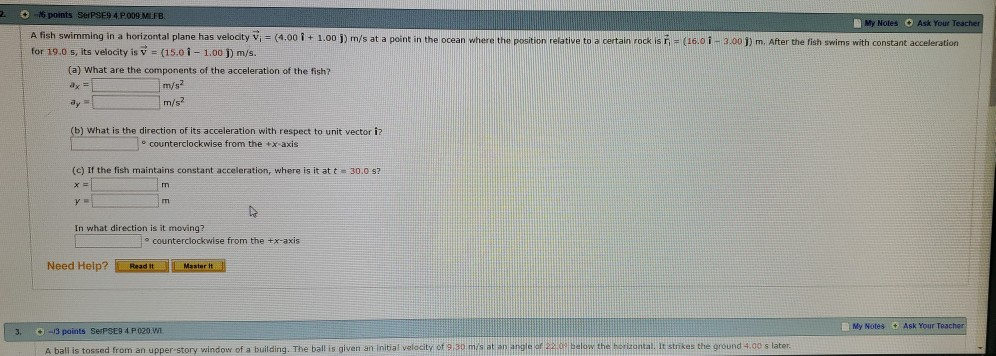2O6 points SerPSE9 4.P.009 MLFB My Notes Ask Your h swimming in a horizontal plane has velocity Vi (4.00 i + 1.00 j) m/s at a point in the ocean where the position relative to a certain rock isi- (16.01 -3.00 1) m. After the fish swims with constant acceleration for 19.0 s, its velocity is v (15.01-1.00 j) m/s. (a) What are the components of the acceleration of the fish? m/s2 m/s2 b) What is the direction of its...